# Triple

(diff) ← Older revision | Latest revision (diff) | Newer revision → (diff)

monad, on a categoryA monoid in the category of all endomorphism functors on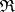. In other words, a triple on a categoryis a covariant functorendowed with natural transformations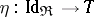and(heredenotes the identity functor on) such that the following diagrams are commutative: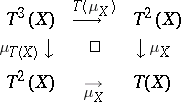A triple is sometimes called a standard construction, cf. .

For any pair of adjoint functorsand(see Adjoint functor) with unit and co-unit of adjunction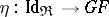and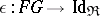, respectively, the functor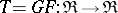endowed withandis a triple on. Conversely, for any triplethere exist pairs of adjoint functorsandsuch that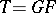, and the transformationsandare obtained from the unit and co-unit of the adjunction in the manner described above. The different such decompositions of a triple may form a proper class. In this class there is a smallest element (the Kleisli construction) and a largest element (the Eilenberg–Moore construction).

## Contents

### Examples.

1) In the category of sets, the functor which sends an arbitrary set to the set of all its subsets has the structure of a triple. Each setis naturally imbedded in the set of its subsets via singleton sets, and to each set of subsets ofone associates the union of these subsets.

2) In the category of sets, every representable functor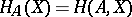carries a triple: The mapping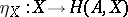associates to eachthe constant function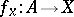with value; the mapping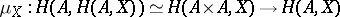associates to each function of two variables its restriction to the diagonal.

3) In the category of topological spaces, each topological group, with unit, enables one to define a functor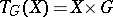that carries a triple: Each elementis taken to the elementand the mappingis defined by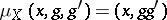.

4) In the category of modules over a commutative ring, each (associative, unital)-algebragives rise to a triple structure on the functor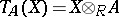, in a manner similar to Example 3).

How to Cite This Entry:
Triple. Encyclopedia of Mathematics. URL: http://encyclopediaofmath.org/index.php?title=Triple&oldid=16681
This article was adapted from an original article by M.Sh. Tsalenko (originator), which appeared in Encyclopedia of Mathematics - ISBN 1402006098. See original article